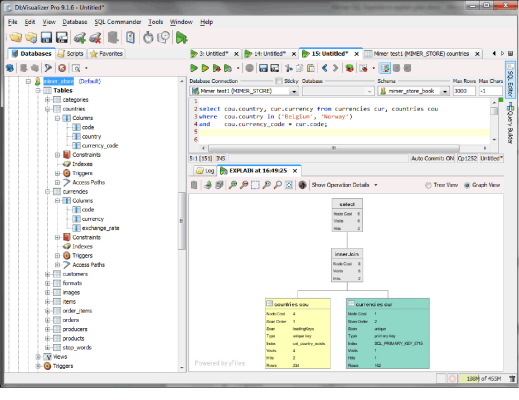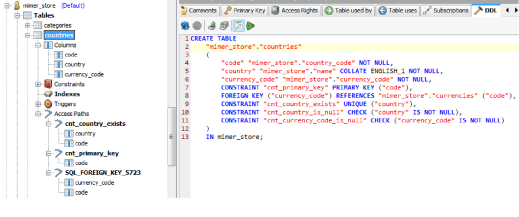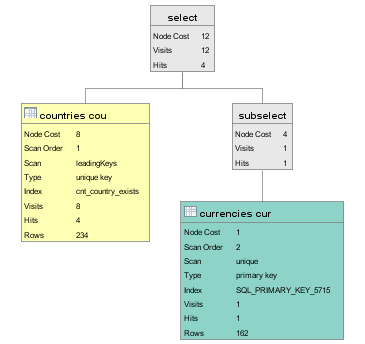Mimer SQL Explain

The Mimer SQL server contains a highly advanced SQL optimizer. The optimizer performs numerous transformations and computes the most efficient access path to get the query results.

It is possible to view the results of the optimization process to help in the construction of efficient queries. In the bsql command line utility (aka. Batch SQL), the optimizer output may be viewed in its raw format. The output is xml-based.

It is also possible to view a graphical output of the same data in the DbVisualizer Pro front-end. (Note that this functionality is not available in the version bundled with the Mimer SQL distribution.) Download a DbVisualizer Pro trial license and try it out! (https://www.dbvis.com/download/)

Here is some reference material about explain output followed by some sample queries.

Node name

Explanation

select

The SQL statement is a select statement

insert

The SQL statement is an insert statement

update

The SQL statement is an update statement

delete

The SQL statement is a delete statement

tempTable

The optimizer has decided to use a temporary table for the results thus far in the query.

union

The optimizer is performing a union all of two or more result sets (i.e. concatenating the results without temporary table). An SQL UNION operation is typically translated to a tempTable containing a union.

subselect

A subselect node is a subquery such as an exists, a scalar subquery, or a quantified predicate (IN-clause etc.). A subquery will be executed for each row found in the outer table(s).

constSubselect

A constSubselect node is a subquery that has no dependencies to outer tables and returns zero or one row. Examples of this are non-correlated exists and scalar subqueries. These are only executed once and the result is reused for each row found in the outer table(s).

innerJoin

The innerJoin node is used when two tables are joined. Only rows that match the join condition are returned.

outerJoin

The outerJoin node is used for an outer join query. When there is no match in the outer join, null values are returned.

crossJoin

This is a join where there are no join conditions between two tables. Every row in the first table will match all rows returned by the second table. The query may be lacking appropriate conditions when this occurs. Double check that your query returns the desired results.

table

This node is used to access the contents of a single table and/or index.

The following attributes are used:

Attribute

Used by

Explanation

name

table

Table name including alias used in SQL statement.

order

table

Scan order of the table/index in the query.

index

table

Here we see the name of the access path picked by the optimizer. The name depends on the type, either name of the index or the constraint. When a base table is read the primary key or internal key is always used.

scan

table

- sequential: this is a complete table scan. In general, they should be avoided as with more data in the table the query will take longer to execute.

- trailingKeys: Means there are conditions on the second or later columns in the index. Unless all previous columns contain very few data values, this will be an expensive scan.

- leadingKeys: One or several of the first columns in the index have conditions on them. Usually a good access path.

- unique: The entire key is specified. Always a very good access path.

type

table

- Primary key.

- Index created with a create index statement.

- Foreign key index.

- Unique constraint index.

- Internal key. This is a generated key for tables without a primary key defined.

indexLookupOnly

table

When accessing an index, first the index is read and then the base table. However, if an SQL statement only uses column values that are present in the index then indexLookupOnly can be used. In this case only the index is read and no corresponding base table lookup is made.

Please note that when a primary key or internal key is used, only the base table is read even though this option is not set.

cost

All nodes

The estimated cost for the accessing the table.

hits

All nodes

The number of hits that will be returned.

visits

All nodes

The number or rows that the system will read from a table or temporary table. When an index is accessed this includes both index table lookup and base table lookup (unless indexLookupOnly is used in which case the base table is never accessed). For parent nodes (such as a join node) this is the accumulated value for underlying accesses.

tempWrites

tempTable

Number of rows written to temporary table.

rows

table

This is the number of rows in the table. This is the value from the last time update statistics was run on the table. If there are no statistics collected for table the optimizer will look in the table to estimate the number of rows.

Join

Let us start with a simple query. It uses the Mimer sample database (see The EXLOAD program.) Login as MIMER_STORE with password GoodiesRUs if you want to try it:

select cou.country, cur.currency from currencies cur, countries cou

where  cou.country in ('Belgium', 'Norway')

and    cou.currency_code = cur.code;

The query returns which currency is used in Belgium and Norway. The explain plan in DbVisualizer looks as follows:The diagram is read starting in the lower left corner and then working your way up to the right. If in doubt, check the scan order field to see the execution order. The same query in bsql is shown here. Note the command set explain on is given first to see the explain plan:

SQL> set explain on;

SQL> select cou.country, cur.currency from currencies cur, countries cou

SQL& where  cou.country in ('Belgium', 'Norway')*

SQL& and    cou.currency_code = cur.code;

Start of explain result

<select cost="6" hits="2" visits="6">

<innerJoin cost="6" hits="2" visits="6">

<table name="countries cou" order="1" index="cnt_country_exists"

cost="4" hits="2" visits="4" rows="234"/>

<table name="currencies cur" order="2" index="SQL_PRIMARY_KEY_5715"

scan="unique" type="primary key"

cost="1" hits="1" visits="1" rows="162"/>

</innerJoin>

</select>

End of explain result

country

currency

================================================

Belgium

Euros

===

Norway

Norwegian Kroner

===

2 rows found

Let us examine the explain output in some detail. Note that the XML contains exactly the same information as is used by DbVisualizer to display the explain graph.

The join order picked by the optimizer is to start with the countries table. This table is then joined with the currencies table.

<table name="countries cou" order="1" index="cnt_country_exists"

scan="leadingKeys" type="unique key" cost="4" hits="2" visits="4" rows="234"/>

Order 1 shows that the countries table is read first. The unique key cnt_country_exists index is used to scan the table. We have a condition on the first column in the index (cou.country = 'Belgium') which is why the scan is "leadingKeys". In DbVisualizer you can see all the columns of cnt_country_exists under Access Paths:The index cnt_country_exists has both the country column and the primary key code column. The visit count is 4 because two rows are read in the index, and two rows from the base table. This will result in a hit-count of 2 rows. The statistics indicates there are 234 rows in the countries table.

Let us now examine the join-node:

<innerJoin cost="6" hits="2" visits="6">

<table name="countries cou" order="1" index="cnt_country_exists" scan="leadingKeys"

type="unique key" cost="4" hits="2" visits="4" rows="234"/>

<table name="currencies cur" order="2" index="SQL_PRIMARY_KEY_5715" scan="unique"

type="primary key" cost="1" hits="1" visits="1" rows="162"/>

</innerJoin>

The join node contains the cost of processing the two tables. The number of visits and hits are as follows:

Visits (6) = visits in countries (4) + hits in countries (2) * visits in currencies (1)

Hits (2) = hits in countries (2) * hits in currencies (1)

When there are no temporary tables involved the cost is equal to the total number of visits.

Let us see what would happen if we were to force the opposite join order. This is done by using the {order} clause in the from-list:

SQL> set explain on;

SQL> select cou.country, cur.currency

SQL& from {order} currencies cur, countries cou

SQL& where  cou.country in ('Belgium', 'Norway')

SQL& and    cou.currency_code = cur.code;

Start of explain result

<select cost="486" hits="162" visits="486">

<innerJoin cost="486" hits="162" visits="486">

<table name="currencies cur" order="1" index="SQL_PRIMARY_KEY_5715"

scan="sequential" type="primary key"

cost="162" hits="162" visits="162" rows="162"/>

<table name="countries cou" order="2" index="SQL_FOREIGN_KEY_5723"

cost="2" hits="1" visits="2" rows="234"/>

</innerJoin>

</select>

End of explain result

This was clearly a bad idea. We now got a sequential scan of the currencies table. Since we only had a join condition (cou.currency_code = cur.code) on this table which cannot be evaluated until we read both tables we get 162 hits. The countries table now uses the foreign key index to find the values. The inner join cost was:

Visits (486) = visits in currencies (162) + hits in currencies (162) * visits in countries (2)

Hits (162) = hits in currencies (162) * hits in countries (1)

Inner join, outer join and cross join are all computed in this way.

Temporary Tables

There are several different types of temporary tables depending on which operation is being handled. For example distinct, group by and order by. Tables used for order by and group by are both inserted to and read. This is seen as both a write count and a visit count. Distinct temporary tables are only used to avoid duplicates and never have to be read. In this case we have a write count, but no visit count. It is a bit more expensive to perform write operations than read operations. The optimizer currently uses a factor of ~1.4 to estimate the cost.

Let us look at an example:

SQL> set explain on;

SQL> select cou.country from currencies cur, countries cou

SQL& where    cou.currency_code = cur.code

SQL& and cur.currency in ('Swiss Francs', 'Pulas', 'Czech Korony')

SQL& order by cou.country;

Start of explain result

<select cost="175" hits="3" visits="171" tempWrites="3">

<tempTable cost="172" class="TempTableOrderBy" hits="3" visits="3" tempWrites="3">

<innerJoin cost="168" hits="3" visits="168">

<table name="currencies cur" order="1" index="SQL_PRIMARY_KEY_5715"

scan="sequential" type="primary key"

cost="162" hits="3" visits="162" rows="162"/>

<table name="countries cou" order="2" index="SQL_FOREIGN_KEY_5723"

cost="2" hits="1" visits="2" rows="234"/>

</innerJoin>

</tempTable>

</select>

End of explain result

As can be seen the optimizer estimates that 3 rows will be both written and read from the temporary table. The cost (172) = innerjoin cost (168) + tempWrites (3) * 1.4. In the next level we add the cost for reading (visits="3") so the total cost is 175.

Subqueries

There are three variations of optimization of subqueries that are important to understand:

The simplest one is where the subquery is executed once for each row of the outer query.

Sometimes it is possible to execute the subquery as a join with the outer table(s). In this case the subquery can occur somewhere in the join order. Depending on the actual conditions a temporary table may be needed to eliminate duplicates in this case.

If the subquery is not correlated, i.e. has no conditions that relate to the outer query and only return zero or one rows, then the query can be executed once and for all and the result is then reused as the outer tables are processed.

We will look at examples of the above three cases.

select cou.country from countries cou

where country in ('Andorra','Angola','Anguilla','Antigua and Barbuda')

and   cou.currency_code in (select cur.code from currencies cur

where exchange_rate > 0.3);For each hit in the countries table we run the subselect. In the query the optimizer has used the primary key to look up the corresponding row in the currencies table. The subselect is evaluated 4 times and each has a cost of 1.

We will now take a look at a query where the subquery participates in the outer query join.

SQL> set explain on;

SQL> select cur.currency

SQL& from currencies cur

SQL& where exists (select 1 from countries c

SQL&               where c.country in ('Sweden' ,'Japan')

SQL&               and c.currency_code = cur.code);

Start of explain result

<select cost="9" hits="2" visits="6" tempWrites="2">

<tempTable cost="9" class="TempTableJoinBySubselect" tempWrites="2">

<innerJoin cost="6" hits="2" visits="6">

<table name="countries c" order="1" index="cnt_country_exists"

cost="4" hits="2" visits="4" rows="234"/>

<table name="currencies cur" order="2" index="SQL_PRIMARY_KEY_5715"

scan="unique" type="primary key"

cost="1" hits="1" visits="1" rows="162"/>

</innerJoin>

</tempTable>

</select>

End of explain result

In the explain output the exists now participates as part of the join with the currencies table. An extra temporary table is in this query needed to eliminate duplicates.

And finally the third type is the constant subquery.

SQL> set explain on;

SQL> select cur.currency

SQL& from  currencies cur

SQL& where cur.exchange_rate < (select exchange_rate

SQL&                            from currencies xcur, countries cou

SQL&                            where  cou.country = 'Belgium'

SQL&                            and    cou.currency_code = xcur.code);

Start of explain result

<select cost="165" hits="54" visits="165">

<constSubselect cost="3" hits="1" visits="3">

<innerJoin cost="3" hits="1" visits="3">

<table name="countries cou" order="1" index="cnt_country_exists"

cost="2" hits="1" visits="2" rows="234"/>

<table name="currencies xcur" order="2" index="SQL_PRIMARY_KEY_5715"

scan="unique" type="primary key"

cost="1" hits="1" visits="1" rows="162"/>

</innerJoin>

</constSubselect>

<table name="currencies cur" order="3" index="SQL_PRIMARY_KEY_5715"

scan="sequential" type="primary key"

cost="162" hits="54" visits="162" rows="162"/>

</select>

End of explain result

The select using currencies and countries is a scalar subselect that only returns a single value. There are no references to the outer table currencies cur. Therefore the constSubelect node can be used. It is executed only once, and then the result is used for each row in the currencies cur table. In the query above the cost is much cheaper than evaluating the subquery for each row in currencies cur (instead of a cost of 165 we would get 660 = 165 + 165 * 3).

Union

Let us look at an example of how union costs are computed:

SQL> set explain on;

SQL> select c.category from categories c

SQL& union

SQL& select f.format from formats f;

Start of explain result

<select cost="30" hits="13" visits="16" tempWrites="10">

<tempTable cost="30" class="TempTableUnion" visits="3" tempWrites="10">

<union cost="13" hits="13" visits="13">

<table name="formats f" order="1" index="fmt_primary_key"

scan="sequential" type="primary key"

cost="10" hits="10" visits="10" rows="10"/>

<table name="categories c" order="2" index="ctg_primary_key"

scan="sequential" type="primary key"

cost="3" hits="3" visits="3" rows="3"/>

</union>

</tempTable>

</select>

End of explain result

In a union duplicates are eliminated. This can be seen as a tempTable node. The cost of the union is the cost of each branch and then the temporary table handling. Remember that writes cost an extra ~1.4 in the cost. It can be noted that the optimizer has reordered the union branches because the select from the categories tables only returns distinct values because there is a unique index on the category column. When the last union branch is distinct (i.e. that branch alone does not return any duplicate values), the system will only check if the value has been seen in earlier union branches. This can be seen as tempWrites="10" (from the first union branch) and visits="3" to check that the value has not occurred before. The total cost (30) = union cost (13) + tempWrites 10 * 1.4 + temp table visits (3).

The optimizer does not know anything about the actual content of the two columns. However, the SQL programmer may know that the values in the two tables are distinct. If that is the case the query can be rephrased as:

SQL> set explain on;

SQL> select c.category from categories c

SQL& union all

SQL& select f.format from formats f;

Start of explain result

<select cost="13" hits="13" visits="13">

<union cost="13" hits="13" visits="13">

<table name="categories c" order="1" index="ctg_primary_key"

scan="sequential" type="primary key"

cost="3" hits="3" visits="3" rows="3"/>

<table name="formats f" order="2" index="fmt_primary_key"

scan="sequential" type="primary key"

cost="10" hits="10" visits="10" rows="10"/>

</union>

</select>

End of explain result

The change is that UNION ALL is used instead of UNION. This will, of course, result in a more efficient query as no temporary table handling is needed. The cost has subsequently dropped from 30 to 13!